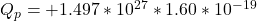## What is the total electric charge of 2.5 kg of (a) electrons and (b) protons?

Question

What is the total electric charge of 2.5 kg of (a) electrons and (b) protons?

in progress 0
2 months 2021-07-24T01:30:11+00:00 1 Answers 3 views 0

a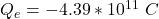b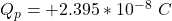Explanation:

Generally the number of electron in the given mass is  mathematically evaluated as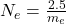Here  m_e is the mass of electron with value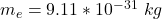=>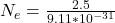=>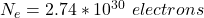The total electric charge is mathematically represented as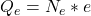Here e is the charge on a single electron with value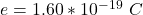So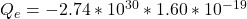The  negative sign is because we are considering electron

Generally the number of  protons in the given mass is  mathematically evaluated as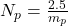Here  m_p is the mass of electron with value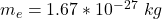=>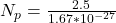=>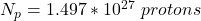The total electric charge is mathematically represented as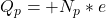Here p is the charge on a single proton with value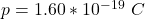So